## Important Questions of Characteristics of Electric Motors:

### Problems of Characteristics of Electric Motors:

1.A 50 hp, 220 V, 1200 rpm dc shunt motor has an efficiency of 85% at full load. The shunt field resistance is 110Ω and the armature circuit resistance is 0.06Ω. This motor is required to drive a load requiring 120% of the full load motor torque at a speed of 550 rpm. Determine the additional armature resistance required to operate the motor.

2.A 50 hp, 220 V, 1200 rpm shunt motor has an armature resistance of 0.06Ω. At rated speed and output the motor takes a current of 180 A. The field current is 2 A. The field flux is reduced to 70% of its original value by using a field rheostat while the torque remains the same. Find the new speed and efficiency, assuming that the rotational losses vary as the square of speed.

3.The armature of a dc shunt motor has a resistance of 0.08Ω. The armature current drawn by the motor at a speed of 1400 rpm is 190 A. The motor drives a load having a torque speed characteristic given by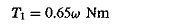where ω is rad/s. If the rated voltage of the motor is 230 V determine the torque and speed of the motor.

4.(a) Determine the additional armature resistance required to reduce the speed to 600 rpm in Problem 3. (b) Determine also the voltage to be applied to the motor for the conditions of 4(a). Assume the field is separately excited from a 230 V supply.

5. A 600 V, 50 hp, 600 rpm dc series motor has an armature and series field resistance of 0.2Ω. The full load current of the motor is 215 A. The armature voltage is varied to control the speed of the motor. Determine the voltage required to reduce the speed to 450 rpm if the torque driven by the motor is given by the relation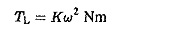where ω is the speed in rad/s.

6. A series motor having a combined series and field resistance of 2Ω draws a current of 8 A from a 240 V supply and runs at a speed of 400 rpm. (a) Determine the torque developed, assuming a linear magnetisation curve. (b) Determine the value of resistance to be connected in series with the armature circuit if the speed has to be reduced to 200 rpm keeping the torque at its value in (a). (c)What would be the speed of the motor to develop (3/4)th of the torque in (a) if the resistance connected is double the value in (b)?

7. The magnetisation curve of a dc series motor, obtained by separately exciting the field is given in the following table, at 800 rpm. The combined armature and field resistance is 0.2Ω when connected as a series motor. Rheostatic braking is employed to limit the speed of the motor to 400 rpm against a load torque of 15 Nm. Determine the current drawn by the motor. The moment of inertia of the motor is 100 kgm2. Determine also the braking time.

8. A 220 V dc shunt motor with constant field drives a load whose torque is proportional to square of the speed. When running at 800 rpm, it takes an armature current of 40 A. Find the speed at which the motor runs if a resistance of 12Ω is connected in series with the armature. The armature resistance may be taken as 1Ω.

9. A 200 V dc shunt motor has a no-load speed of 700 rpm with rated field current. The resistance of the armature is 0.9Ω. The rated current of the motor is 20 A. A resistance of 6Ω in series and 3Ω in parallel, is connected with it. Determine the current drawn and speed at full load torque.

10. A dc shunt motor takes 70 A from a 220 V supply and runs at 1000 rpm; when delivering an output of 11 kW. The armature circuit resistance is 0.15Ω. Determine the additional resistance required in the armature to reduce the speed to 800 rpm, if

• the load torque is proportional to the square of the speed
• the load torque is constant
• Determine the loss in the resistance in both the cases.

11. The armature resistance of a dc shunt motor is 0.6Ω. The motor draws an armature current of 180 A at a speed of 1250 rpm and operates on a supply system of 220 V. It drives a load torque having a characteristic.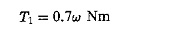where ωis in rad/s. Determine the torque and speed of the motor. Determine also the torque and speed when the load has a characteristic.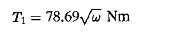12. A 220 V dc shunt motor runs at 700 rpm with full field. The armature resistance is 0.5Ω. The armature current required to give rated torque is 20 A. The speed of the motor is controlled by resistances connected in series and parallel with the armature, equal to 6Ω and 5Ω respectively. Determine the speed-torque characteristic. What current would the motor-draw to develop rated torque? What is the efficiency?

13. A 20 hp, 1200 rpm, 220 V shunt motor has an armature resistance of 0.2Ω and field resistance of 124Ω. The series and shunt resistances connected are 0.05 and 2Ω respectively. Find the speed of the motor required to develop the rated torque. Assume its efficiency as 85%.

14. Consider a 20 hp, 1200 rpm, 220 V shunt motor having an armature resistance of 0.2Ω and field resistance of 124Ω. An extra resistance of 10Ω is connected in the field circuit. Find the speed at which the machine runs while developing the rated torque. Assume the efficiency of the motor without extra field resistance as 85%.

15. A series motor having a combined series field and armature resistance of 1.5Ω drives a fan load having a torque speed characteristic given by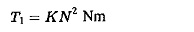where N is in rpm. At 220 V the motor runs at 350 rpm taking a current of 8 A. The speed of the fan has to be increased to 500 rpm by increasing the armature voltage. Determine the voltage and current at the new speed. Neglect saturation.

16. A series motor working on a 500 V system runs at a rated speed of 1000 rpm and develops rated torque with a current of 95 A. The armature resistance is 0.15Ω and series field resistance is 0.05 ohm.

Assuming that the magnetic circuit is unsaturated, determine:          .

• the speed of the armature when the supply current is 40 A and the armature voltage is 450 V.
• the speed when the motor develops 50% of the full load torque with a diverter resistance of 0.05Ω connected in parallel with the field. The supply voltage is 500 V.
• The machine is supplied with rated voltage and has a resistance of 0.15Ω in series with the armature. Determine the speed when the motor develops 75% of rated torque.

17. A 250 V shunt motor with a constant field current of 3.2 A runs at a no-load speed of 600 rpm. The rotational inductance is 0.8 H armature resistance is 0.5Ω and the system inertia is 5 kgm2. Determine the braking times and currents if the motor is to be retarded to (a) 300 rpm (b) standstill, when braked with an external resistance of 4.5Ω (i) dynamically (ii) by reverse-current.

18. The 250 V shunt motor problem 1 runs at no-load at a speed of 600 rpm. Find the braking time for the motor to be retarded to 300 rpm regeneratively (a) by halving the armature voltage, (b) by reducing the field circuit resistance to half its value at 600 rpm. The field inductance is 4.5 H. Neglect armature inductance.

19. A 240 V dc shunt motor has an armature resistance of 0.06Ω and an emf constant of 2.2 V rad/s. The motor runs at a speed of 1000 rpm. It is overhauling a load with a torque of 200 Nm

• Calculate the resistance to be inserted to lower the load at 1000 rpm.
• If regenerative braking is employed, determine the minimum speed at which the motor can hold the load.

20. A 200 V, 25 kW dc shunt motor runs at its rated speed of 1500 rpm. Reverse current braking is employed. The armature resistance is 0.15Ω and the efficiency is 87%. Determine

• the resistance required in series with the armature to limit the initial braking current to 2.5 times the rated value.
• the initial braking torque.
• the armature current and torque when the speed has fallen to 500 rpm.

21. A series motor having a combined field and armature resistance of 1.5Ω between the terminals drives a load for which the torque is proportional to square of the speed. At 250 V the motor draws a current of 15 A and runs at 400 rpm. If the speed is to be decreased to 200 rpm, determine the value of resistance required in the armature circuit for the limiting cases of (a) linear variation of flux with field current, (b) constant flux due to saturation.

22. A series motor has an unsaturated field having armature and field resistances of 0.5 and 0.7Ω respectively. It drives a load for which the torque is proportional to the cube of the speed. The motor, while operating on a 250 V supply, takes 45 A and runs at 300 rpm. Determine the voltage required to raise the speed to 450 rpm.

23. A series motor having a combined series and field resistance of 1.5Ω drives a load whose speed-torque characteristic is given by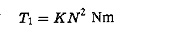where N is in rpm. At 200 V it runs at a speed of 300 rpm taking a current of 10 A. The speed of the load has to be increased to 500 rpm; determine the voltage and current at the new speed by (a) neglecting saturation (b) assuming the flux is almost constant.

24. A 400 V, 4 pole, 50 Hz motor delivers its rated load of 10 kW at 1450 rpm. It has a rotor resistance per phase of 0.3Ω. If an extra resistance of 2Ω is connected in every phase, determine the speed of the motor. Assume constant torque.

25. A 3-phase induction motor has a full load slip of 4%. It has resistance and standstill reactances per phase of 0.02 and 0.1Ω respectively. The motor drives a constant torque load. The speed has to be reduced to 50% of rated speed.

• Determine the percentage reduction in stator voltage.
• Repeat the problem if Td α ω2

26. The speed of a slip ring induction motor is varied by means of a resistance in the rotor circuit. The motor drives a load whose torque is proportional to square of the rotor-speed. The details of the motor are: 3-phase, 440 V, 50 Hz, 12 pole, 492 rpm, stator and rotor connected in star. The motor delivers a power of 200 kW at rated speed. What resistance must be added to the rotor circuit to reduce the speed to 200 rpm? Assume the rotor resistance is 0.02Ω/phase. The stator to rotor turns ratio is 1.2. Neglect stator impedance and rotational losses of the motor.

27. The rotor resistance and standstill reactance of a wound rotor induction motor are 0.15Ω and 1.0Ω per phase respectively. The motor has a turns ratio of 1.2 The applied voltage per phase of the 8 pole stator is 220 V at 50 Hz. Determine additional rotor resistance to produce maximum torque at (a) starting, (b) a speed of 350 rpm.

28. (a) Show that in a 3-phase induction motor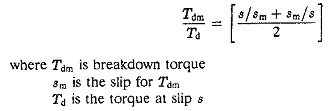(b) A 3-phase, 50 Hz induction motor has a starting torque of 1.8 times full load torque and a breakdown torque of 2.5 times full load torque, while operating on its rated voltage rated frequency mains. Determine the speeds for maximum as well as rated torque.

29. A 3-phase, 4 pole, 50 Hz induction motor has a full load slip of 5% while operating at rated voltage and rated frequency. The rotor resistance and standstill reactance are r2 = 0.02Ω and x2 = 0.15Ω respectively. Find the speed of the motor when the voltage is reduced by 30% and the torque delivered by the motor is the rated full load torque.

30. A 3-phase 220 V, 50 Hz, six pole induction motor has the following parameters: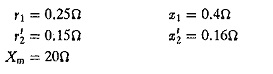For a slip of 3%, determine the stator current, output power, torque and efficiency of the motor. Assume constant losses = 350 W.

31. A 3-phase, 8 pole, 50 Hz induction motor has rotor parameters of r2 = 0.15Ω and x2 = 0.6Ω.

Neglecting stator parameters and rotational losses, determine the speed of the motor when a rated voltage of 234 V is applied. The load torque is proportional to square of the rotor angular velocity. The constant of proportionality is 0.0136. If the voltage applied is reduced by 25%, determine the speed.

32. A 3-phase, 4 pole, 440 V, 50 Hz slip ring induction motor has a full load slip of 3%. The stator to rotor turns ratio = 1.8. The rotor resistance and standstill reactance are 0.2Ω and 0.8Ω respectively. Determine

• the maximum torque
• the slip and speed at which maximum torque occurs
• the resistance to be connected in the rotor circuit to give a starting torque of 8 times maximum torque.

33. The shunt motor of Example 35 runs at a no-load speed of 1000 rpm. The motor is braked by means of plugging. Determine the braking time and current to reduce the speed to (a) 500 rpm and (b) standstill.

Scroll to Top